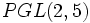# Group cohomology of symmetric group:S5

View group cohomology of particular groups | View other specific information about symmetric group:S5

## Classifying space and corresponding chain complex

The homology and cohomology groups are the same as the respective homology and cohomology groups of the configuration space of five unordered points in a countable-dimensional real projective space. For more on the topological perspective, see configuration space of unordered points of a countable-dimensional real vector space on the Topology Wiki.

## Family contexts

Family name Parameter values General discussion of group cohomology of family
symmetric group$S_n$ of degree$n$$n = 5$ group cohomology of symmetric groups
projective general linear group of degree two over a finite field of size$q$$q = 5$, i.e., field:F5, so the group is$PGL(2,5)$ group cohomology of projective general linear group of degree two over a finite field

## GAP implementation

### Computation of homology groups

The homology groups for trivial group action on the integers can be computed using the Hap package (if the package is installed but not automatically loaded, load it using LoadPackage("hap");), specifically its GroupHomology function. The function returns a list of numbers which are the orders of cyclic groups whose external direct product is the desired homology group.

#### First homology group

The first homology group, which is also the abelianization, can be computed as follows:

```gap> GroupHomology(SymmetricGroup(5),1);
[ 2 ]```

This says that$H_1(S_5;\mathbb{Z}) = \mathbb{Z}/2\mathbb{Z}$.

#### Second homology group

The second homology group, which is also the Schur multiplier, can be computed as follows:

```gap> GroupHomology(SymmetricGroup(5),2);
[ 2 ]```

This says that$H_2(S_5;\mathbb{Z}) = \mathbb{Z}/2\mathbb{Z}$.

#### First few homology groups

```gap> List([1..8],i -> [i,GroupHomology(SymmetricGroup(5),i)]);
[ [ 1, [ 2 ] ], [ 2, [ 2 ] ], [ 3, [ 2, 4, 3 ] ], [ 4, [ 2 ] ],
[ 5, [ 2, 2, 2 ] ], [ 6, [ 2, 2 ] ], [ 7, [ 2, 2, 4, 3, 5 ] ],
[ 8, [ 2, 2, 2 ] ] ]```